# Circuits Practice Flashcards

Set Details Share
created 5 years ago by ssdesa_100
3,491 views
updated 5 years ago by ssdesa_100
Page to share:
Embed this setcancel
COPY
code changes based on your size selection
Size:
X

1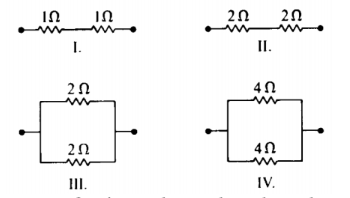Multiple Correct. Which arrangements of resistors shown above have the same resistance between the terminals? Select two answers
(A) I
(B) II
(C) III
(D) IV

A, D

2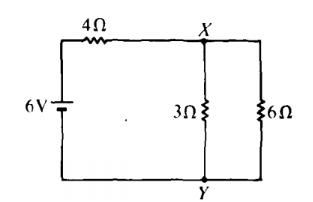In the circuit shown above, what is the value of the potential difference between points X and Y if the 6–volt
battery has no internal resistance?
(A) 2 V (B) 3 V (C) 4 V (D) 6V

(A)

3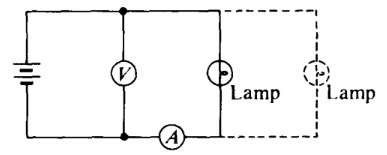How would the ammeter reading change when another lamp is connected in parallel with the first lamp as shown by the dashed lines?
(A) increases, because the current through the ammeter splits to feed both branches
(B) remains the same, because the ammeter measures the current provided by the battery
(C) decreases, because the resistance of the circuit is increased
(D) remains the same, because energy is conserved in the circuit

(A)

4How would the voltmeter reading change when another lamp is connected in parallel with the first lamp as shown by the dashed lines?
(A) decreases, because the current is split between the two branches
(B) remains the same, because charge is conserved in the circuit
(C) increases, because the resistance of the circuit is increased
(D) remains the same, because energy is conserved in the circuit

(D)

5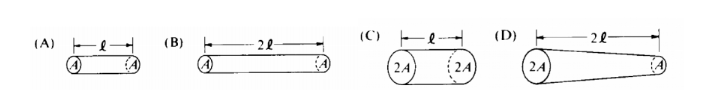The four resistors shown below have the lengths and cross–sectional areas indicated and are made of material
with the same resistivity. Which has the greatest resistance?

(A)
(B)
(C)
(D)

(B)

6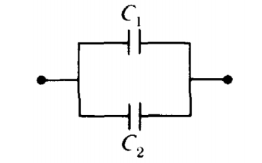Two capacitors are connected in parallel as shown above. A voltage V is applied to the pair. What is the ratio of charge stored on C1 to the charge stored on C2, when C1 = 1.5C2 ?

(A) 2/3
(B) 1
(C) 3/2
(D) 9/4

(C)

7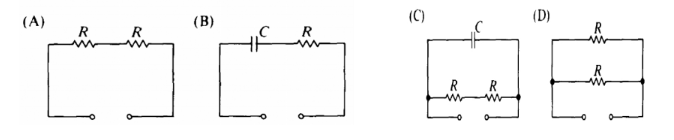The five incomplete circuits below are composed of resistors R, all of equal resistance, and capacitors C, all of
equal capacitance. A battery that can be used to complete any of the circuits is available.

Into which circuit should the battery be connected to obtain the greatest steady power dissipation?
(A) A
(B) B
(C) C
(D) D

(D)

8The five incomplete circuits below are composed of resistors R, all of equal resistance, and capacitors C, all of
equal capacitance. A battery that can be used to complete any of the circuits is available.

Which circuit will retain stored energy if the battery is connected to it and then disconnected?

(A) A
(B) B
(C) C
(D) D

(B)

9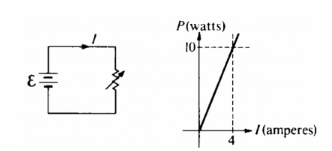The circuit shown above left is made up of a variable resistor and a battery with negligible internal resistance. A graph of the power P dissipated in the resistor as a function of the current I supplied by the battery is given above right. What is the emf of the battery?
(A) 0.025 V
(B) 2.5 V
(C) 6.25 V
(D) 40 V

(B)

10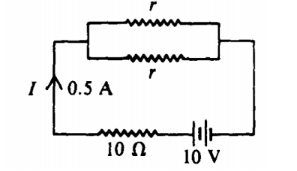In the circuit shown above, the value of r for which the current I is 0.5 ampere is
(A) 1 ꭥ
(B) 5 ꭥ
(C) 10 ꭥ
(D) 20 ꭥ

(D)

11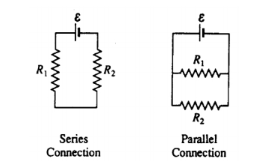In the diagrams above, resistors R1 and R2 are shown in two different connections to the same source of emf E that has no internal resistance. How does the power dissipated by the resistors in these two cases compare?
(A) It is greater for the series connection, because the current is not split.
(B) It is greater for the series connection, because the equivalent resistance is greater
(C) It is greater for the parallel connection, because the total current is greater.
(D) It is greater for the parallel connection, because both resistors have the same voltage.

(C)

12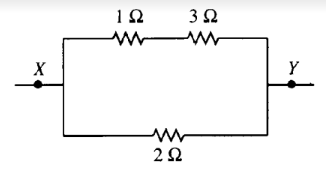The diagram above shows part of a closed electrical circuit. When there is a steady current in the circuit, the amount of charge passing a point per unit of time is
(A) greater in the 1 ꭥ resistor than in the 3 ꭥ resistor
(B) greater in the 1 ꭥ resistor than in the 2 ꭥ resistor
(C) greater in the 2 ꭥ resistor than in the 3 ꭥ resistor
(D) greater at point X than at point Y

(C)

13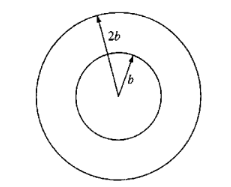Two concentric circular loops of radii b and 2b, made of the same type of wire, lie in the plane of the page, as shown above. The total resistance of the wire loop of radius b is R. What is the resistance of the wire loop of radius 2b?
(A) R/2
(B) R
(C) 2R
(D) 4R

(C)

14

The total capacitance of several capacitors in parallel is the sum of the individual capacitances for which of the following reasons?
(A) The charge on each capacitor depends on its capacitance, but the potential difference across each is the same.
(B) The charge is the same on each capacitor, but the potential difference across each capacitor depends on its
capacitance.
(C) Capacitors in a circuit always combine like resistors in series.
(D) The parallel combination increases the effective separation of the plates.

(A)

15

A wire of length L and radius r has a resistance R. What is the resistance of a second wire made from the same material that has a length L/2 and a radius r/2?
(A) 4R
(B) 2R
(C) R
(D) R/2

(B)

16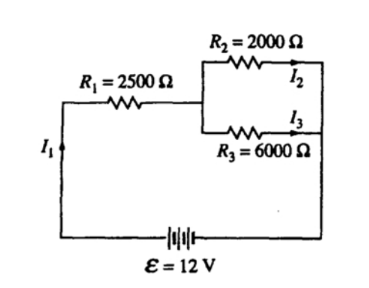Which current is greater I1 or I2?
(A) I1 is greater, because it has more resistance.
(B) I2 is greater, because it has less resistance.
(C) I1 is greater, because of charge conservation.
(D) I2 is greater, because of energy conservation.

(C)

17What is the current I1?
(A) 1 mA
(B) 3 mA
(C) 4 mA
(D) 12 mA

(B)

18Which of the following changes would increase the value of I1?
(A) Remove R3 and the branch containing it.
(B) Replace R2 with another 6000 Ohm resistor.
(C) Add an 8000 Ohm resistor in parallel with R2 and R3.

(C)

19

A 60-W incandescent bulb and a 13.3-Watt compact fluorescent light are both plugged into a 110-volt household circuit and lit. Which bulb has the greater resistance?
(A) Neither, since they are connected across the same potential difference.
(B) The 60-W bulb, because it draws more current.
(C) The 13.3-W bulb, because it uses less power
(D) The 13.3-W bulb, because it is more efficient.

(B)

20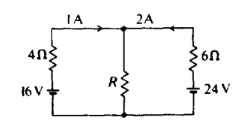In the circuit shown above, what is the resistance R?
(A) 3 ꭥ
(B) 4 ꭥ
(C) 6 ꭥ
(D) 12 ꭥ

(B)

21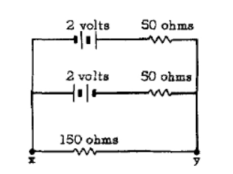In the circuit shown above, the current in each battery is 0.04 ampere. What is the potential difference between the points x and y?
(A) 8 V
(B) 2 V
(C) 0 V
(D) 4 V

(C)

22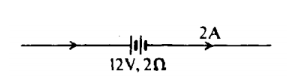A 12–volt storage battery, with an internal resistance of 2, is being charged by a current of 2 amperes as shown in the diagram above. Under these circumstances, a voltmeter connected across the terminals of the battery will read
(A) 8 V
(B) 10 V
(C) 12 V
(D) 16 V

(D)

23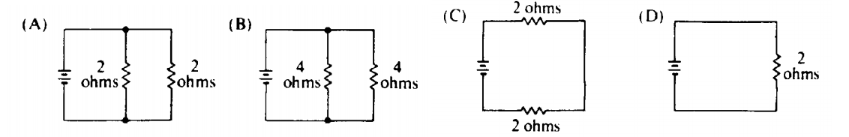The batteries in each of the circuits shown above are identical and the wires have negligible resistance.

In which circuit is the current furnished by the battery the greatest?
(A) A
(B) B
(C) C
(D) D

(A0

24The batteries in each of the circuits shown above are identical and the wires have negligible resistance.

In which circuit is the equivalent resistance connected to the battery the greatest?
(A) A
(B) B
(C) C
(D) D

(C)

25The batteries in each of the circuits shown above are identical and the wires have negligible resistance.

Which circuit dissipates the least power?
(A) A
(B) B
(C) C
(D) D

(C)

26

When two identical parallel–plate capacitors are connected in series, which of the following is true of the equivalent capacitance?
(A) It depends on the charge on each capacitor.
(B) It depends on the potential difference across both capacitors.
(C) It is larger than the capacitance of each capacitor.
(D) It is smaller than the capacitance of each capacitor.

(C)

27

The emf of a battery is 12 volts. When the battery delivers a steady current of 0.5 ampere to a load, the potential difference between the terminals of the battery is 10 volts. What terminal voltage would you expect if the battery were connected to a load with less resistance?
(A) 12 V
(B) 10 V
(C) Between 10 and 12 V
(D) Less than 10 V

(D)

28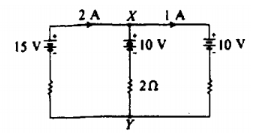In the circuit shown above, the emf's of the batteries are given, as well as the currents in the outside branches and the resistance in the middle branch. What is the magnitude of the potential difference between X and Y?
(A) 4 V
(B) 8 V
(C) 10 V
(D) 12 V

(D)

29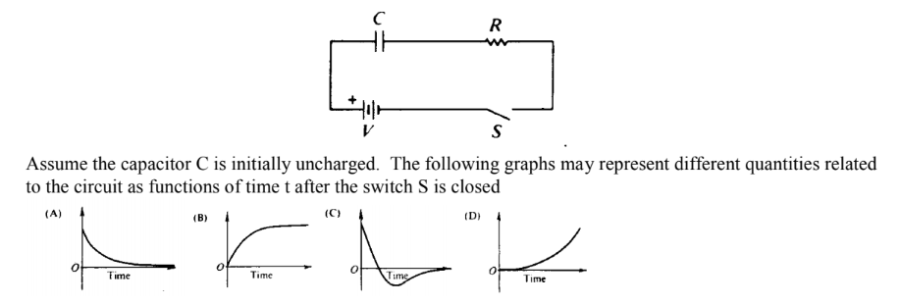Which graph best represents the voltage versus time across the resistor R?
(A) A
(B) B
(C) C
(D) D

(A)

30Which graph best represents the current versus time in the circuit?
(A) A
(B) B
(C) C
(D) D

(A)

31Which graph best represents the voltage across the capacitor versus time?
(A) A
(B) B
(C) C
(D) D

(B)

32

A wire of resistance R dissipates power P when a current I passes through it. The wire is replaced by another wire with resistance 3R. The power dissipated by the new wire when the same current passes through it is
(A) P/9
(B) P/3
(C) P
(D) 3P

(D)

33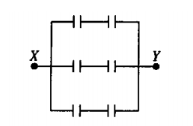The diagram above represents a circuit of six 2–microfarad capacitors. What potential difference must be applied between points X and Y so that the charge on each plate of each capacitor will have magnitude 6
microcoulombs?
(A) 3V
(B) 6 V
(C) 9 V
(D) 18 V

(B)

34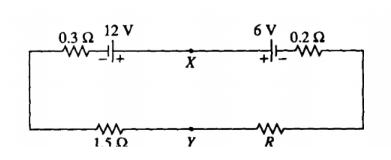In the circuit above, the emf's and the resistances have the values shown (0.3 Ω, 0.2 Ω and 1,5 Ω) . The current I in
the circuit is 2 amperes.

34. The resistance R is
(A) 1 ꭥ
(B) 2 ꭥ
(C) 3 ꭥ
(D) 4 ꭥ

(A)

35In the circuit above, the emf's and the resistances have the values shown (0.3 Ω, 0.2 Ω and 1,5 Ω) . The current I in
the circuit is 2 amperes.

The potential difference between points X and Y is
(A) 1.2 V
(B) 6.0 V
(C) 8.4 V
(D) 10.8 V

(C)

36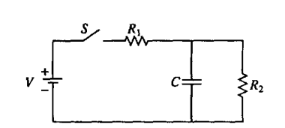In the circuit shown above, the battery supplies a constant voltage V when the switch S is closed. The value of the capacitance is C, and the value of the resistances are R1 and R2. In the time after the switch is closed, the current supplied by the battery is
(A) constant, because batteries always provide constant current
(B) constant, because the capacitor is a open circuit and doesn’t affect the two resistors in series
(C) decreasing, because current initially flows to the capacitor
(D) decreasing, because it becomes zero when the capacitor is full

(C)

37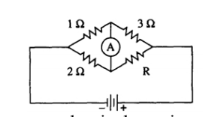If the ammeter in the circuit above reads zero, what is the resistance R ?
(A) 1.5 ꭥ
(B) 2 ꭥ
(C) 4 ꭥ
(D) 6 ꭥ

(D)

38If the current through the ammeter is to flow toward the bottom of the page, how must the resistance R compare to the value found in #37?
(A) it must be larger, so that the voltage across R increases
(B) it must be larger, so that the equivalent resistance of the circuit increases
(C) it must be smaller, so that the voltage across R decreases
(D) it must be smaller, so that the equivalent resistance of the circuit decreases

(A)

39

A resistor R and a capacitor C are connected in series to a battery of terminal voltage V0. Which of the following equations relating the current I in the circuit and the charge Q on the capacitor describes this circuit?

(A) V0 + QC – I2R = 0
(B) V0 – Q/C – IR = 0
(C) V0 2 – Q2/2C – I2R = 0
(D) V0 – CI – I2R = 0

(B)

40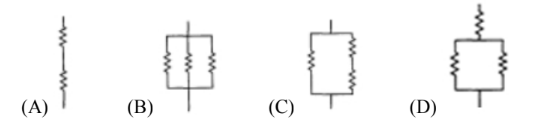Which of the following combinations of 4 resistors would dissipate 24 W when connected to a 12 Volt battery?

(A)
(B)
(C)
(D)

(D)

41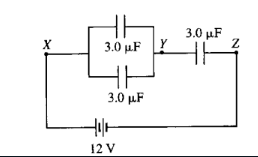Three identical capacitors, each of capacitance 3.0 F, are connected in a circuit with a 12 V battery as shown above. The potential difference between points Y and Z is
(A) 6 V, because all capacitors will have the same potential difference
(B) 8 V, because charge is conserved in the set of wires containing point Y.
(C) 6 V, because charge is conserved in the set of wires containing point Y.
(D) 8 V, because all capacitors have the same charge.

(B)

42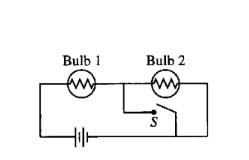The circuit in the figure above contains two identical lightbulbs in series with a battery. At first both bulbs glow with equal brightness.
When switch S is closed, which of the following occurs to the bulbs?
Bulb I Bulb 2
(A) Goes out Gets brighter
(B) Gets brighter Goes out
(C) Gets brighter Gets slightly dimmer
(D) Gets slightly dimmer Gets brighter

(B)

43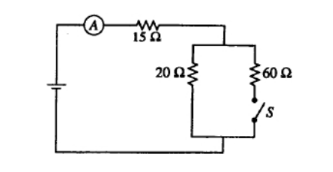When the switch S is open in the circuit shown above, the reading on the ammeter A is 2.0 A. When the switch is closed, the reading on the ammeter is
(A) doubled
(B) increased slightly but not doubled
(C) the same
(D) decreased slightly

(B)

44

Two conducting cylindrical wires are made out of the same material. Wire X has twice as much resistance than wire Y. Which of the following could be true?
(A) Wire X is twice the diameter of wire Y.
(B) Wire X is twice as long and twice the diameter of wire Y.
(C) Wire Y is twice as long and twice the diameter of wire X.
(D) Wire Y is twice as long as wire X.

(C)

45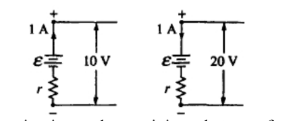The figures above show parts of two circuits, each containing a battery of emf ε and internal resistance r. The current in each battery is 1 A, but the direction of the current in one battery is opposite to that in the other. If the potential differences across the batteries' terminals are 10 V and 20 V as shown, what are the values of ε and r?
(A) E = 5 V, r = 15 Ω
(B) E =10 V, r = 100 Ω
(C) E = 15 V, r = 5 Ω
(D) E = 20 V, r = 10 Ω

(C)

46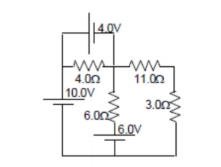What is the current through the 6.0 ꭥ resistor shown in the accompanying circuit diagram? Assume all batteries have negligible resistance.
(A) 0 A
(B) 0.40 A
(C) 1.3 A
(D) 1.5 A

(A)

47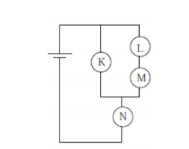Rank the current through the bulbs.
(A) K > L > M > N
(B) L = M > K = N
(C) L > M > K > N
(D) N > K > L = M

(D)

48Bulb K burns out. Which of the following statements is true?
(A) Only bulb N goes out.
(B) Bulb N becomes brighter.
(C) The brightness of bulb N remains the same.
(D) Bulb N becomes dimmer but does not go out.

(D)

49Bulb M burns out. Which of the following statements is true?
(A) Only bulb M goes out.
(B) Bulb N goes out but at least one other bulb remains lit.
(C) The brightness of bulb N remains the same.
(D) Bulb N becomes dimmer but does not go out.

(D)

50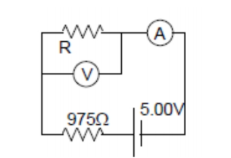The voltmeter in the accompanying circuit diagram has internal resistance 10.0 kꭥ and the ammeter has internal resistance 25.0 ꭥ. The ammeter reading is 1.00 mA. The voltmeter reading is most nearly:
(A) 1.0 V
(B) 3.0 V
(C) 4.0 V
(D) 5.0 V

(C)

51

When two resistors, having resistance R1 and R2, are connected in parallel, the equivalent resistance of the combination is 5 ꭥ. Which of the following statements about the resistances is correct?
(A) Both R1 and R2 are greater than 5 ꭥ.
(B) Both R1 and R2 are equal to 5 ꭥ.
(C) Both R1 and R2 are less than 5 ꭥ.
(D) The sum of R1 and R2 is 5 ꭥ.

(A)

52

When a single resistor is connected to a battery, a total power P is dissipated in the circuit. How much total power is dissipated in a circuit if n identical resistors are connected in series using the same battery? Assume the internal resistance of the battery is zero.
(A) n2P
(B) nP
(C) P/n
(D) P/n2

(C)

53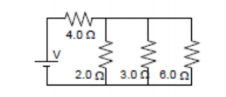In the accompanying circuit diagram, the current through the 6.0–ꭥ resistor is 1.0 A. What is the power supply voltage V?
(A) 10 V
(B) 18 V
(C) 24 V
(D) 30 V

(D)

54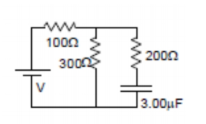In the circuit diagrammed above, the 3.00–ꭥF capacitor is fully charged at 18.0 ꭥC. What is the value of the power supply voltage V?
(A) 4.40 V
(B) 6.00 V
(C) 8.00 V
(D) 10.4 V

(C)

55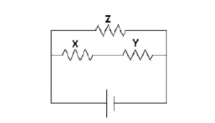Given the simple electrical circuit above, if the current in all three resistors is equal, which of the following statements must be true?
(A) X, Y, and Z all have equal resistance
(B) X and Y have equal resistance
(C) X and Y added together have the same resistance as Z
(D) X and Y each have more resistance than Z

(C)

56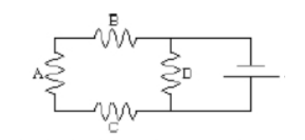If all of the resistors in the above simple circuit have the same resistance, which would dissipate the greatest power?
(A) resistor A
(B) resistor B
(C) resistor C
(D) resistor D

(D)

57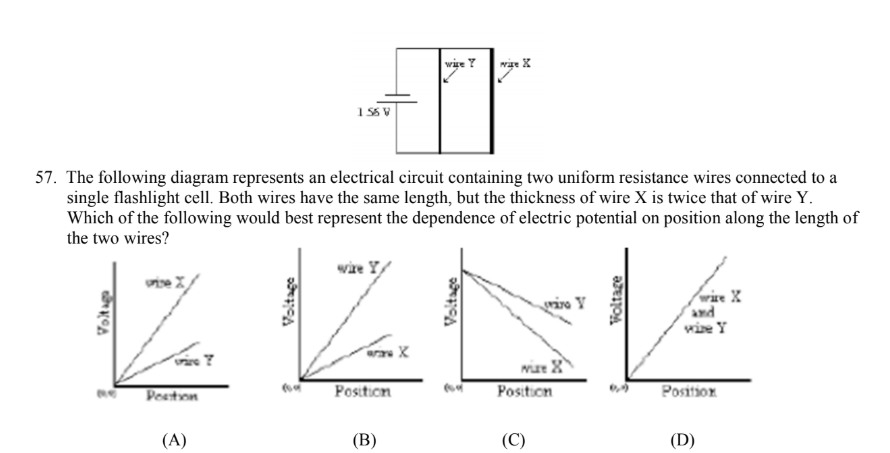The following diagram represents an electrical circuit containing two uniform resistance wires connected to a single flashlight cell. Both wires have the same length, but the thickness of wire X is twice that of wire Y.

Which of the following would best represent the dependence of electric potential on position along the length of the two wires?
(A)
(B)
(C)
(D)

(D)

58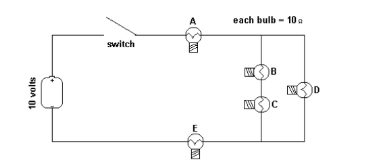Five identical light bulbs, each with a resistance of 10 ohms, are connected in a simple electrical circuit with a switch and a 10 volt battery as shown in the diagram below. Which bulb (or bulbs) could burn out without causing other bulbs in the circuit to also go out?
(A) only bulb D
(B) only bulb E
(C) only bulbs C or D
(D) bulbs B, C, or D

(A)

59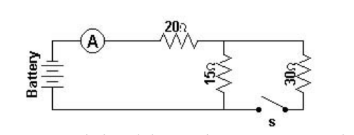With the switch open, what would be the potential difference across the 15 ohm resistor?
(A) 30 V
(B) 40 V
(C) 60 V
(D) 70 V

(A)

60With the switch open, what must be the voltage supplied by the battery?
(A) 30 V
(B) 40 V
(C) 60 V
(D) 70 V

(D)

61When the switch is closed, what would be the current in the circuit?
(A) 1.7 A
(B) 2.0 A
(C) 2.3 A
(D) 3.0 A

(C)

62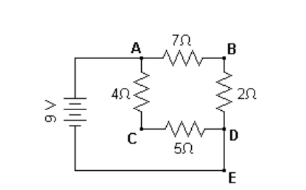How would the current through the 2 ohm resistor compare to the current through the 4 ohm resistor?
(A) twice as large
(B) one–half as large
(C) equally as large
(D) four times as large

(C)

63What would be the potential at point B with respect to point C in the above circuit?
(A) +7 V
(B) +3 V
(C) 0 V
(D) –3 V

(D)

64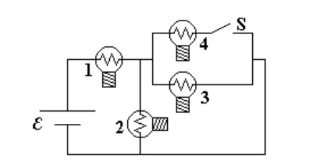A circuit is connected as shown. All light bulbs are identical. When the switch in the circuit is closed
illuminating bulb #4, which other bulb(s) also become brighter?
(A) Bulb #1 only
(B) Bulb #2 only
(C) Bulbs #2 and #3 only
(D) Bulbs #1, #2, and #3

(A)

65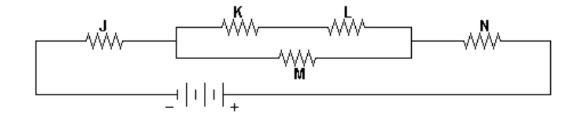Through which resistor(s) would there be the greatest current?
(A) M only
(B) N only
(C) J and N only
(D) K and L only

(C)

66Which resistor(s) have the greatest rate of energy dissipation?
(A) M only
(B) N only
(C) J and N only
(D) K and L only

(C)

67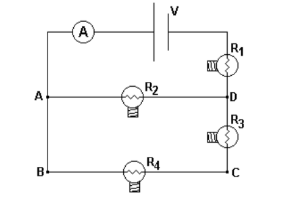The circuit shown has an ideal ammeter with zero resistance and four identical resistance light bulbs which are initially illuminated. A person removes the bulb R4 from its socket thereby permanently breaking the electrical circuit at that point. Which statement is true of the circuit after removing the bulb?
(A) The voltage from B → C increases.
(B) The power supplied by the battery increases
(C) The voltage across R1 increases.
(D) The ammeter reading is unchanged.

(A)

68

A current through the thin filament wire of a light bulb causes the filament to become white hot, while the larger wires connected to the light bulb remain much cooler. This happens because:

(A) the larger connecting wires have more resistance than the filament.
(B) the thin filament has more resistance than the larger connecting wires.
(C) the filament wire is not insulated.
(D) the current in the filament is greater than that through the connecting wires.

(B)

69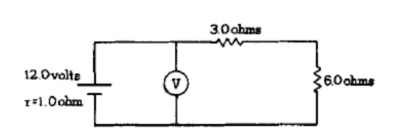In the circuit above the voltmeter V draws negligible current and the internal resistance of the battery is 1.0 ohm. The reading of the voltmeter is
(A) 10.0 V
(B) 10.5 V
(C) 10.8 V
(D) 11.6 V

(C)

70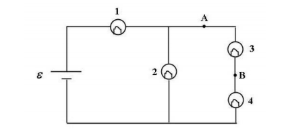For the circuit shown, a shorting wire of negligible resistance is added to the circuit between points A and B. When this shorting wire is added, bulb #3 goes out. Which bulbs (all identical) in the circuit brighten?
(A) Only Bulb 4
(B) Only Bulbs 1 and 4
(C) Only Bulbs 2 and 4
(D) Bulbs 1, 2 and 4

(B)

71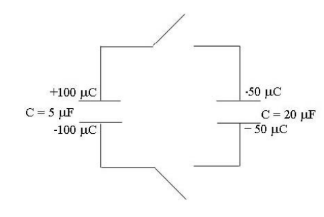For the configuration of capacitors shown, both switches are closed simultaneously. After equilibrium is established, what is
the charge on the top plate of the 5 μF capacitor?
(A) 50 μC
(B) 30 μC
(C) 25 μC
(D) 10 μC

(D)

72

A student wants to make a brighter light bulb. He decides to modify the filament. How should the filament of a light bulb be modified in order to make the light bulb produce more light at a given voltage?
(A) Increase the resistivity only.
(B) Increase the diameter only.
(C) Decrease the diameter only.
(D) Decrease the diameter and increase the resistivity.

(B)

73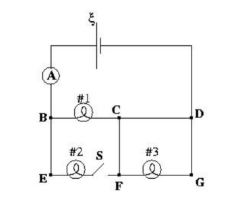For the circuit shown, the ammeter reading is initially I. The switch in the circuit then is closed. Consequently:
(B) The potential difference between E and F increases.
(C) Bulb #3 lights up more brightly.
(D) The power supplied by the battery decreases.

(C)

74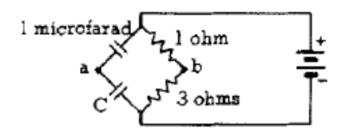In the circuit shown above, the potential difference between points a and b is zero for a value of capacitance C of

(A)

75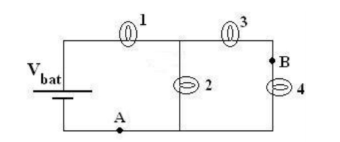For the circuit shown, when a shorting wire (no resistance) connects the points labeled A and B, which of the numbered light bulbs become brighter? Assume that all four bulbs are identical and have resistance R .

(A) Bulb 1 only
(B) Bulb 2 only
(C) Bulb 3 only
(D) Bulbs 1 and 3 only

(D)

76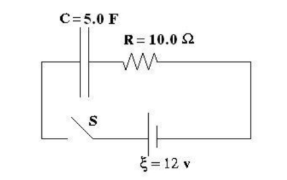For the RC circuit shown, the resistance is R = 10.0 ꭥ, the capacitance is C = 5.0 F and the battery has voltage ξ= 12 volts . The capacitor is initially uncharged when the switch S is closed at time t=0. At some time later, the current in the circuit is 0.50 A. What is the magnitude of the voltage across the capacitor at that moment?
(A) 5 volts
(B) 6 volts
(C) 7 volts
(D) 12 volts

(C)

77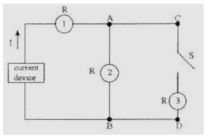In the circuit shown above, a constant current device is connected to some identical light bulbs. After the switch S in the circuit is closed, which statement is correct about the circuit?

(A) Bulb #2 becomes brighter.
(B) Bulb #1 becomes dimmer.
(C) All three bulbs become equally brighter.
(D) The voltage between points C and D is decreased.

(D)

78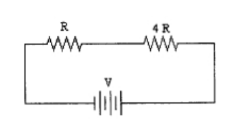Two resistors, one with resistance R and the second with resistance 4R are placed in a circuit with a voltage V.

(A) 4 P
(B) P
(C) 1/2 P
(D) 1/4 P

(A)

79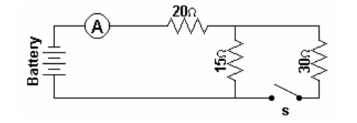A battery, an ammeter, three resistors, and a switch are connected to form the simple circuit shown above. When the switch is closed what would happen to the potential difference across the 15 ohm resistor?
(A) it would equal the potential difference across the 20 ohm resistor
(B) it would be twice the potential difference across the 30 ohm resistor
(C) it would equal the potential difference across the 30 ohm resistor
(D) it would be half the potential difference across the 30 ohm resistor

(C)

80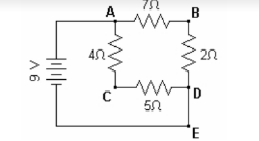What would be the current at point E in the circuit?
(A) 2 amp (B) 4 amp (C) 5 amp (D) 7 amp

(A)

81What would be the potential at point B with respect to point D?
(A) +2 V (B) +4 V (C) +5 V (D) +7 V

(A)

82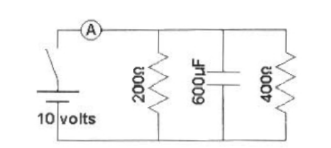Two resistors and a capacitor are connected with a 10 volt battery, a switch and an ideal ammeter to form the simple electrical circuit shown. After the switch is closed and the current in the circuit reaches a constant value, what is the reading on the ammeter in the circuit?
(A) 8.1 × 10–2A
(B) 7.5 × 10–2A
(C) 6.9 × 10–2A
(D) zero

(B)

83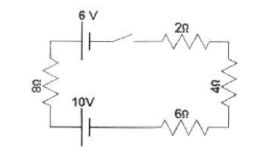When the switch is closed, what would be the current in the circuit shown in the diagram above if the two batteries are opposing one another?
(A) 0.75 A
(B) 0.5 A
(C) 0.3 A
(D) 0.2 A

(D)

84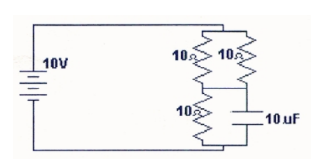The diagram above shows an electrical circuit composed of 3 resistors and 1 capacitor. If each resistor has a resistance of 10 Ω and the capacitor has a value of 10 μF, what would be the charge stored in the capacitor when an EMF of 10 V is maintained in the circuit for a sufficient time to fully charge the capacitor?
(A) 23 μC
(B) 40 μC
(C) 67 μC
(D) 100 μC

(C)

85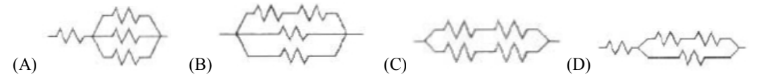Given 4 identical resistors of resistance R, which of the following configurations would have an equivalent resistance of 4/3 R?

(A)
(B)
(C)
(D)

(A)

86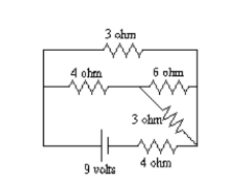What would be the total current being supplied by the battery in the circuit shown above?
(A) 3.0 amperes
(B) 2.0
(C) 1.5 amperes
(D) 1.0 amperes

(C)

87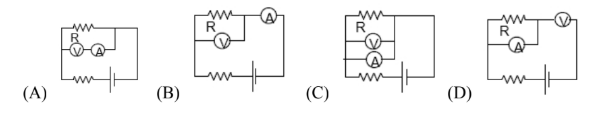Which of the following wiring diagrams could be used to experimentally determine R using Ohm's Law? Assume an ideal voltmeter and an ideal ammeter.

(A)
(B)
(C)
(D)

(B)

88

Closing which of the switches will produce the greatest power dissipation in R2?
(A) S1 only
(B) S2 only
(C) S1 and S2 only
(D) S1 and S3 only

(C)

89

Closing which of the switches will produce the greatest reading on the ammeter?
(A) S1 only
(B) S2 only
(C) S1 and S2
(D) S1 and S3 only

(D)

90

Closing which of the switches will produce the greatest voltage across R3?
(A) S1 only
(B) S2 only
(C) S1 and S2 only
(D) S1 and S3 only

(A)